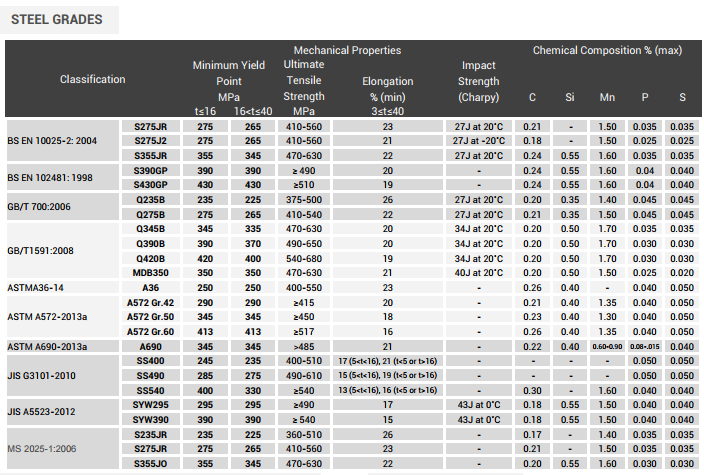Search
•ESC Group

# Understanding Steel Sheet Pile Strength

Steel sheet piles are widely used for earth-retaining structures. These can be designed as cantilever walls for continuous retaining walls and cofferdams, or as braced cuts when dealing with deep excavations for building.A sheet pile wall must resist the following loads once it has been installed at the site:

1. Lateral earth pressures under static conditions

2. Lateral earth pressures due to earthquakes

3. Hydrostatic pressures due to porewater pressure buildup at the retained side

A sheet pile wall is generally idealized as a flexural member, as it mainly resists bending stresses from the above mentioned loads. Its strength can be determined from two components: the geometric property of the sheet pile, and the grade of the material to be used.

We know that for a member subjected to pure bending within the elastic limit, the maximum bending stress of a flexural member is defined as

Where:

σmax = maximum normal stress caused by bending or “flexing”, often referred to as flexural stress

M = bending moment applied to the member, for this case the sheet pile

c = distance from the neutral axis of the member to the extreme tension/compression fiber of the section

I = second moment of area, often referred to as the moment of inertia

Referring to Eq. (1), the adequate member section can be selected by investigating the ratio, I/c. This ratio is defined as the elastic section modulus, S, and is purely dependent on the member’s geometric properties. Substituting S for I/c in Eq. (1), the maximum flexural stress can be defined as follows:

We see in Eq. (2) that smax is inversely proportional to the elastic section modulus, S. This implies that flexural members shall be designed with as large a value of S as is practical . To illustrate this, let’s use a basic example by taking two (2) pieces of wooden beams with rectangular cross sections of the same area, as presented in Figure 1.Figure 1. Two wooden beams of the same area, A (Source: Beer, F.P. Mechanics of Materials, 8th Ed.)

For rectangular beams, the elastic section modulus, S, can be calculated as follows:

We can see from Eq. (3) that for two beams of equal cross-sectional areas (denoted by A), the beam with the larger depth, h, shall have a larger section modulus, and will be more effective in resisting the bending stresses due to the applied loads .This fundamental principle can be applied to other structural shapes as well. For a sheet pile wall, a large portion of the cross-sectional area is located far from the neutral axis of the section. Should a sheet pile section have a large depth, higher values for the moment of inertia, I, and the section modulus, S, are expected. This in turn makes the sheet pile perform better in terms of bending.Apart from the geometric properties of the sheet pile, the grade of the material shall also be considered when selecting the appropriate section. Sheet piles are most often made of structural steel. Compared to other construction materials, steel has yield strength, and is generally ductile in nature. The yield strength of steel varies widely and is dependent on various factors such as the chemical composition and manufacturing process. Material and testing standards also play a critical role in steel yield strength, as some projects would require certain material standards and/or specifications to be met as part of the detailed engineering design.

Going back to Eq. (2), we know that the maximum bending stress decreases as the section modulus increases. If we take the yield strength, Fy, as the maximum bending stress, smax, divided by a safety factor, Ω, Eq. (2) can be expressed as follows:This implies that as the engineer designs a sheet pile wall for a higher steel yield strength, the required section modulus for the project would be less. This translates to a lighter sheet pile section, and design optimization/ value engineering is achieved.